Jump to Section

# Knowing C

## What is C

A, B, and C. That’s it.
Of course, I am kidding. To Understand C, we need have a look on the computer diagram. Here is a brief one.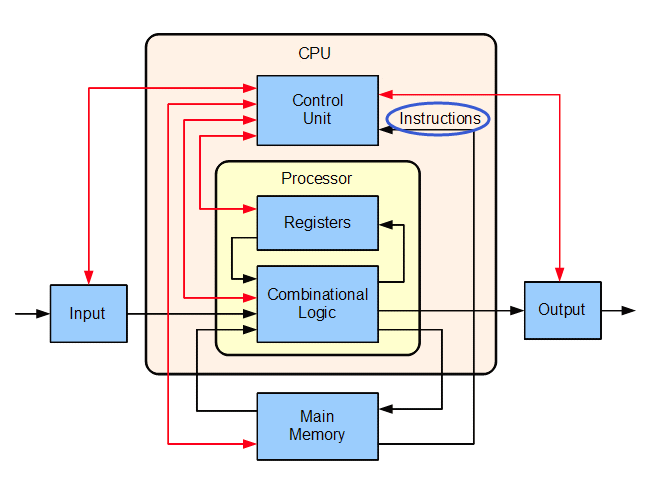A computer is a machine consists of units e.g. memory, CPU, hard-drive. Such a machine won’t do anything unless someone (like you) tell him to do something.

For instance, you tell the machine calculate 1+1. So you need give the instructions. How can you communicate with a machine? You have to use machine language.

C is a high-level language that can be translated to instructions for machines. Such a translator is called compiler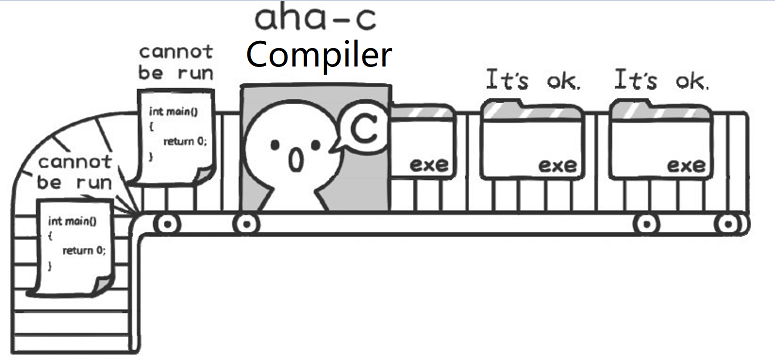## Let the Computer Talk

Why are there computers? Everything the great humans invented is to help us improve our lives. The computer is also a tool to help us. Think about it, if you now want a computer to help you do something, what do you need to do first? Is it necessary to communicate with the computer first? Then communication needs to rely on a language. Body language, Chinese, English, French and German can be used to communicate with people. If you want to communicate with a computer, you need to use a language that the computer can understand. The “C language” we learn is a kind of computer language. In addition to C language, computer languages ​​include C++, Java, and C#. C language is a relatively simple computer language, more suitable for beginners. All computer languages ​​are interlinked. If you can master the C language proficiently, then learning other languages ​​will become easy.

Since the computer is a tool made by humans to help humans, it is obviously very important to let it speak and tell us what it knows.

Let’s solve the first problem: How to make the computer speak?

Recalling that year, when we first came into this world, what was the first sentence we said? It shouldn’t be “Hello!”, “Have you eaten?”… This will scare your parents!

Along with the cry of “wa wa wa”, we came to this wonderful world, and now we also let the computer “cry once”. In this place, there are two ways for the computer to tell us what it wants to say: one is to display it on the monitor screen; the other is to emit sound through a speaker. Just like when we have something to say, one is to write it on paper, the other is to speak it with our mouth. At present, it is more troublesome to let the computer use speakers to output sound, so another method is adopted, which is to use the screen to output “wa wa wa”.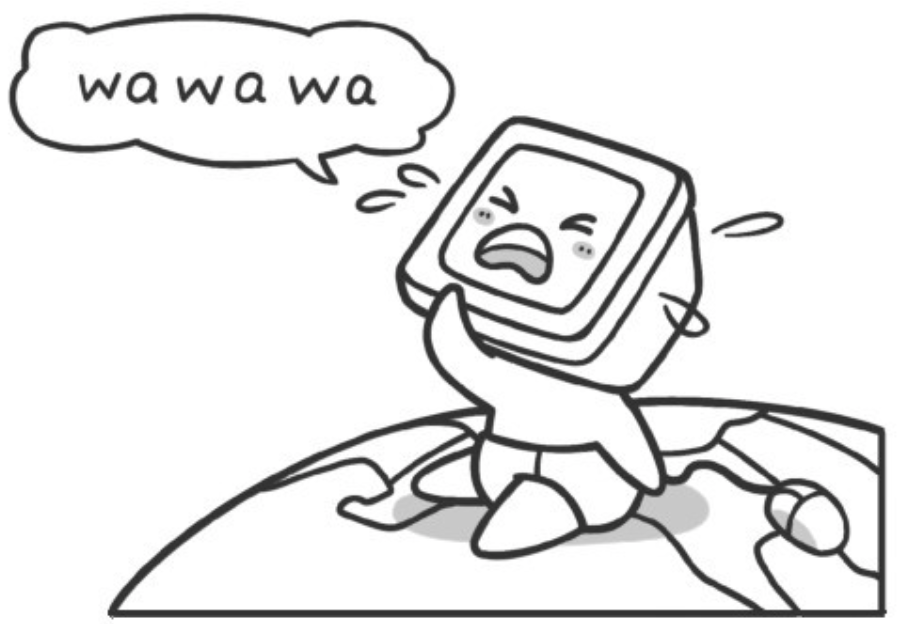``````printf("wa wa wa");
``````

Here is an unfamiliar word called printf. Don’t be frightened by it. You don’t need to figure out what its essential meaning is at present. Just remember that it is the same as “say” in Chinese and “say” in English. However, it is just a word that controls the computer to speak. The () immediately after the printf is like a mouth, and put the content to be said in this “mouth”. There is one more thing to note here. There are "" on both sides of “wa wa wa”, which is what the computer needs to “speak”. Is this very similar to our Chinese? Finally, there must be an end symbol at the end of a sentence. In Chinese, a period is used to indicate the end of a sentence; in English, a period is used to indicate the end of a sentence; in computer languages, a semicolon is used to indicate the end of a sentence.

Note: Every sentence of the computer is a sentence. So, what if you are asked to write a sentence and let the computer say “ni hao”?

``````    printf("ni hao");
``````

Now we let the computer run this statement. It needs to be explained here, just input printf (“ni hao”);, the computer can’t recognize it, you need to add a frame. The complete procedure is as follows:

``````    #include <stdio.h>
#include <stdlib.h>
int main()
{
printf("ni hao");
return 0;
}
``````

Here

``````    #include <stdio.h>
#include <stdlib.h>
int main()
{
return 0;
}
``````

Is must for all C framework, you don’t need to understand it for a while, now know to have the can, later to speak in detail its use.But one thing, we write all similar to the printf statements in the future are to write in {}. You need to let the computer run we just write program. If you let the computer run our writing (in fact we write is a C language program), you need a special software, it is called “C language compiler” , there are many kinds of “C language compiler”, here are a relatively simple software, called “aha C”.

We use Codeblocks. In Windows: codeblocks-20.03mingw-setup.exe from Download Page In Mac: CodeBlocks-13.12-mac.zip from Download Page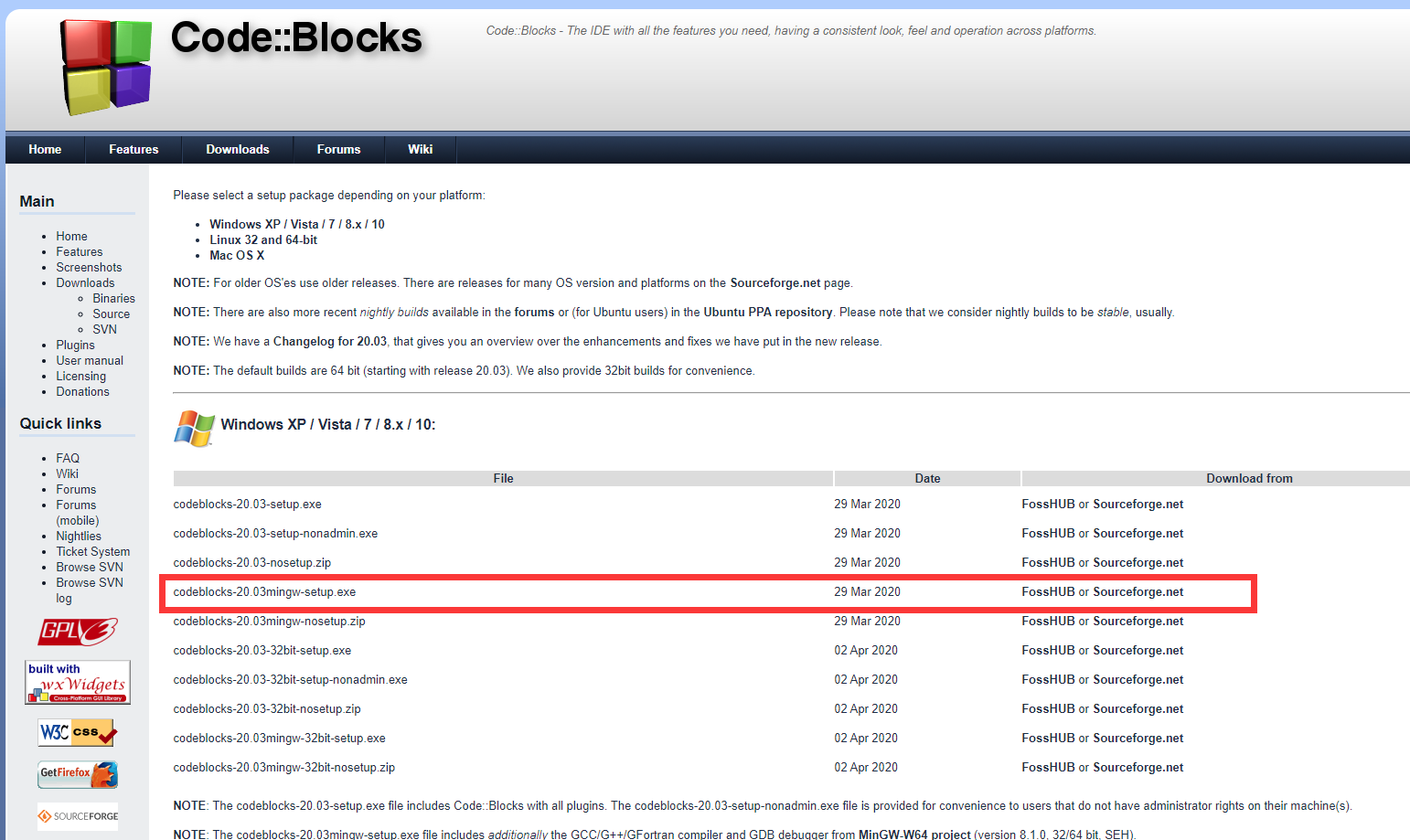Note If you don’t wish install any software, you can run your program online. e.g. online_c_compiler

The Steps for installing can be don everything in default: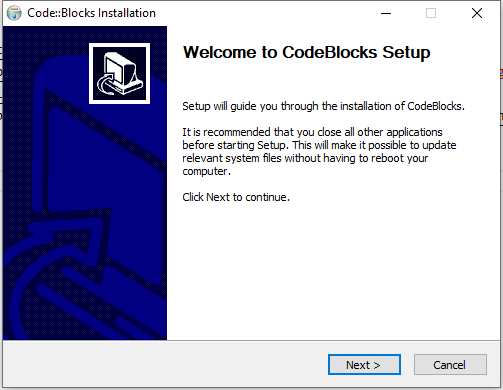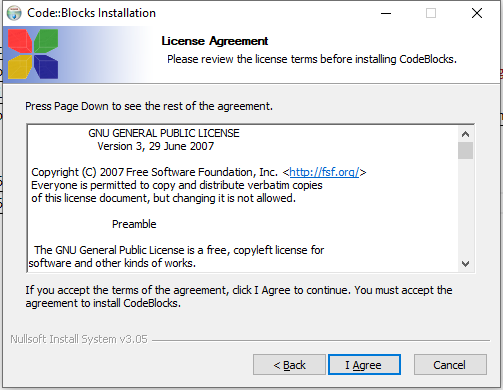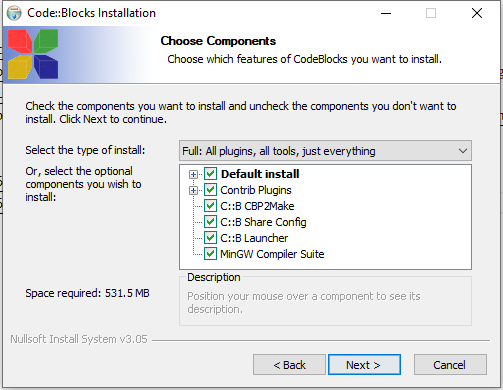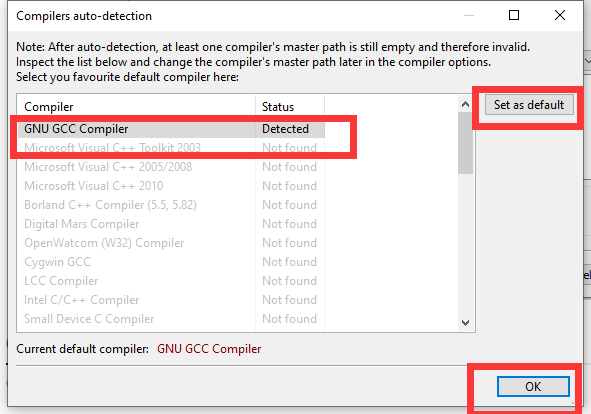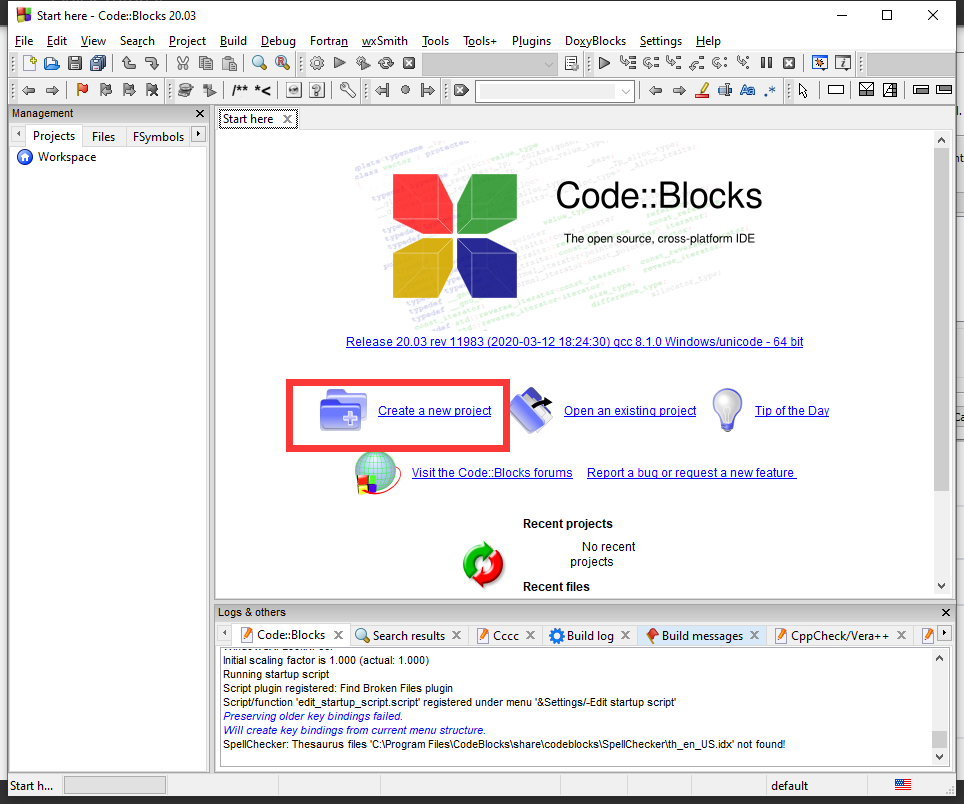Select the “Console Application” and “Go”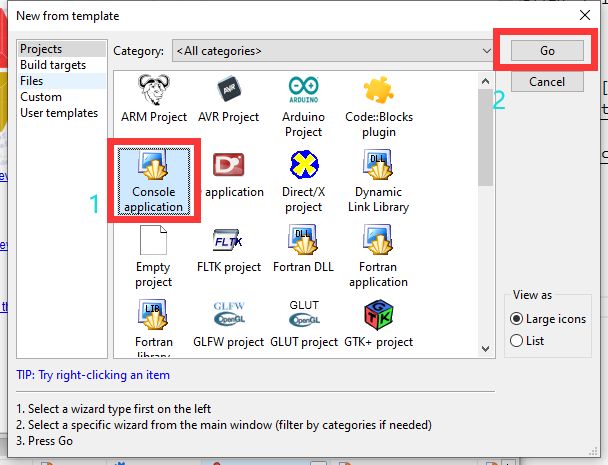Select “C”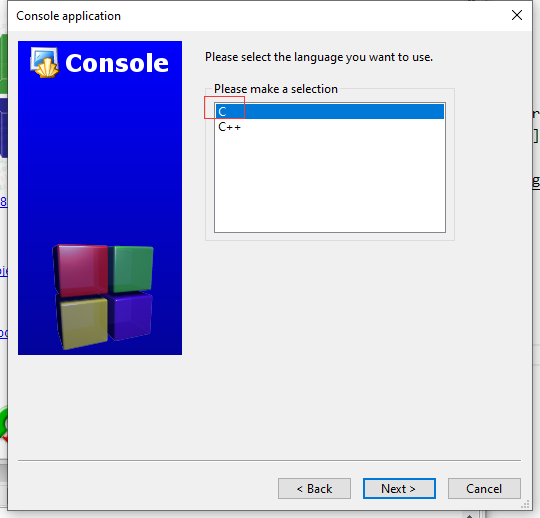Type the project name. We use “aha”; Make sure you give a path. Then finish.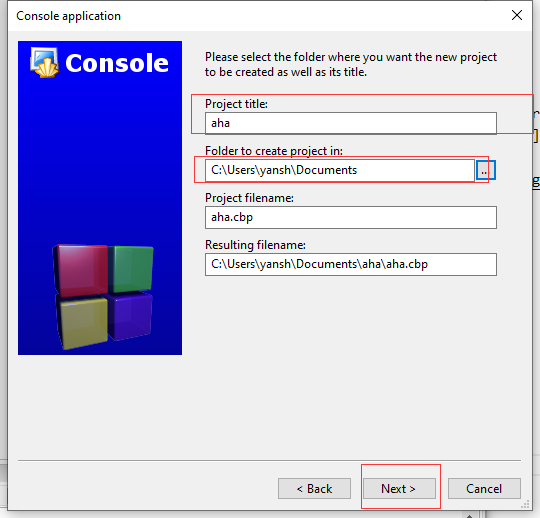Now you have the project. Change “Hello World” to “Aha”.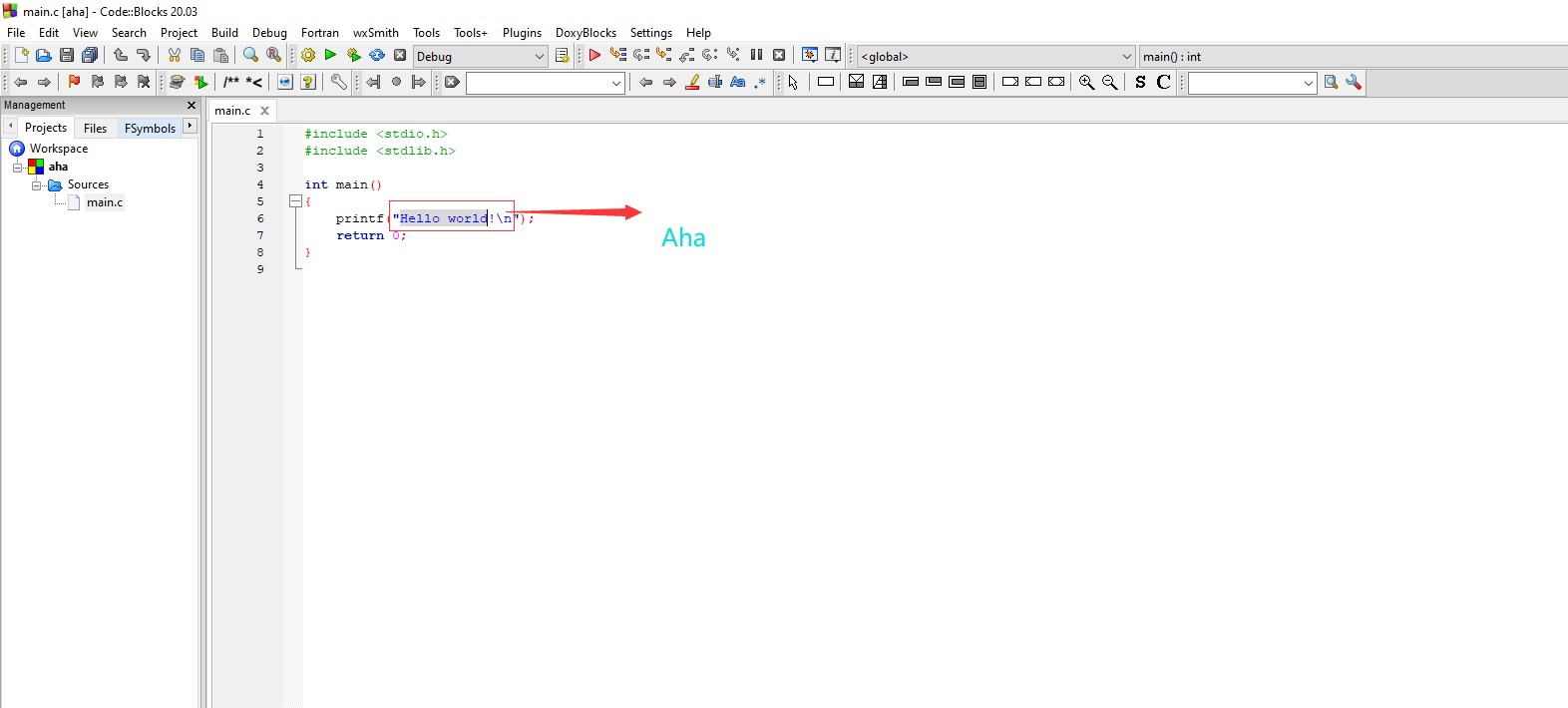Then click “Build and Run”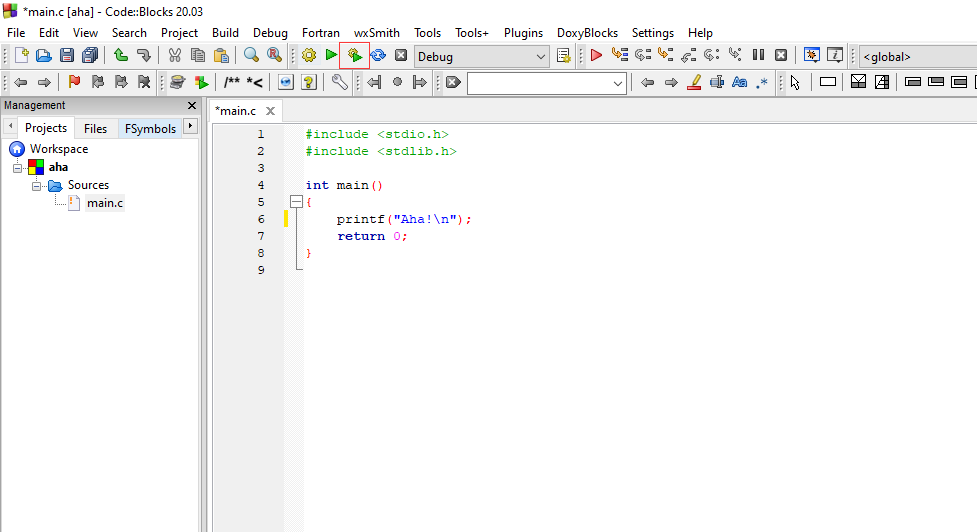As we explained, the build convert your language to machine language. Then we let the machine run the compiled stuff, the run step.

## I need colorful output!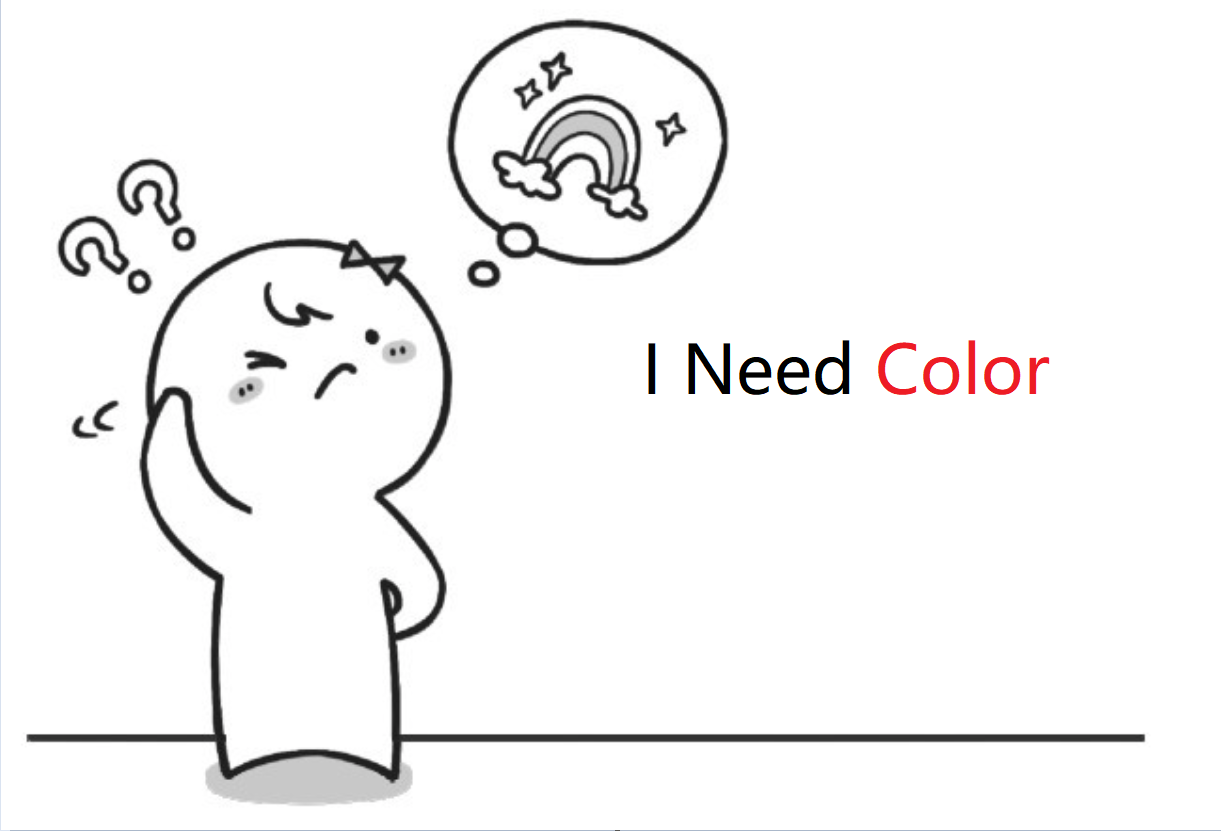In section 2 of this chapter, we learned to let the computer speak printf statements should be used.Can be found, the computer, “said.” the words are all black white, actually the output of the computer can be a color, we together and see it.

Note that this code can only be compiler running under the Windows operating system.If you are using the book recommended by C language software “C” aha, so your code can run successfully for sure.Take a look at how to make color.

Please try to enter the following code and running, see what happens.

``````    #include <stdio.h>
#include <stdlib.h>
int main()
{
system("color 5");
printf("wa wa wa");
system("pause");
return 0 ;
}
``````

The base of color is still dark.The color of the text, however, have been turned into the “purple”, is in the following lines of code.

``````    system("color 5");
``````

In this sentence, “5” on behalf of the “purple”, you can try other Numbers, and see what are the colors. Since the color of the word can change, so the background color can be?Try the following code:

``````    #include <stdio.h>
#include <stdlib.h>
int main()
{
system("color f5");
printf("wa wa wa");
system("pause");
return 0;
}
``````

The above code in front of the original 5 added a f, f here on behalf of the background color is white.

Then set the background color and text color, the color followed by two digits, the first number said the background color, the second number representing text color.If behind the color only added a a number, say only set the text color, background color still use the default color.

To be sure there are actually a number is the hexadecimal, it can only be 0, 1, 2, 3, 4, 5, 6, 7, 8, 9, a, b, c, d, e, f the one.

[aside] “, doesn’t hurt”

Here you learn a new knowledge - into the system.

In modern mathematics, we usually use a decimal, which USES digital 0, 1, 2, 3, 4, 5, 6, 7, 8, 9.After 9 Numbers cannot say, our solution is to use “carry”.Only to 9, for example, Arabic numerals, hence we into one, then the use 0 said, the current is produced by 10 “ten”.Because it is a “dot into one”, so called decimal.

And hexadecimal is “meet hexadecimal”, namely using 0, 1, 2, 3, 4, 5, 6, 7, 8, 9, A, B, C, D, E, F.0 ~ 9 is the same as in the decimal, but the “ten” in capital letters in hexadecimal A said, and so on, “15” in hex F use capital letters to indicate.F is “hex” in the last one, so the number can’t say “16”.So we are just in the decimal said not just into a old way, the current should be represented by 0.“16” in hexadecimal said to 10.27 “in the same way,” said 1 b in hexadecimal.

Many dynasties in ancient China, is made for everyday count hexadecimal, for example, the phrase “well” from allusions hexadecimal;And algorithm is above 2 beads in ancient China, the following 5 beads.If each beads represent Numbers 5 above, the following each beads represent the number 1, then every one of the most important matter is 15, 15 is the maximum cardinality hexadecimal.When using abacus count is greater than 15, we need to “carry” on the abacus.

In fact in our modern daily life, is not all “decimal”, for example, 60 seconds to 1 minute, 60 minutes to 1 hour, is to use the 6 decimal.

## Let the computer do “adding”

Through the previous study, we learned that let the computer speak is to use “printf”, using “printf” can let the computer can say what you like. After learned to “talk”, let’s look at how to make computer do mathematics, first we let the computer to do “addition”, first is “1 + 2”.

Think back to when I was young my father mother is how to teach us is 1 + 2?

Mother said: “the left hand to give you an apple, right hand two apples for you, now there are a few apples?“We quickly thinking, blurted out: “three apples.That’s right!We first use the brain to remember there are a few apples, left hand to use the brain to remember right hand there are a few apples, mother asked when there are few, our brains are very rapid calculation, will just remember to add two Numbers, get the results, finally the calculated results. Analysing, roughly divided into the following five steps.

(1) use the brain to remember the number of apples left; (2) the number of apples with brain remember hand; (3) we will add two Numbers; (4) the results; (5) the results output. During this period, our brains were carried out the following four actions.

(1) the two input are respectively record the number of apples in her left hand and right hand; (2) to store the three values: are respectively record the number of apples in her left hand and right hand, and the result of the combined; (3) conducted a calculation: additive; (4) conducted an output: output the results of the additive. How to let the computer to do addition? Also need more than a few steps.

First of all to solve how to make the computer like our brains to remember a number.

Actually the brains of the computer is like a “skyscraper”, there are a lot of make in the “little house”, the computer put the need to remember that the number of “little house”, a “small house” can only put a number, so that the computer can remember many.Ok, let’s take a look at, specific how to operate.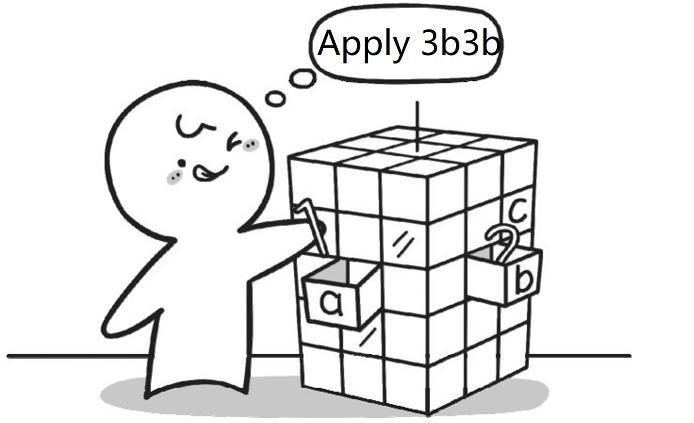The role of the “=” assignment symbol is the equivalent of a hand, put Numbers in the small house.

``````int a, b, c;
``````

This sentence is in the “skyscraper” of the computer application for three small house called a, b and c respectively. (note: there is a space between the int and a, a, b and c respectively use commas between, a semicolon at the end of the end).

Next, we make a little house and the house b to record two Numbers 1 and 2 respectively, specific as follows:

``````a = 1;
b = 2;
``````

Description: here there is a “=”, this is not equal to the number, it is called the number given (also known as assignment), similar to an arrow “please”, meaning is assigned to the content of the “=” the right “=” to the left.For example, the number 1 for a small house, so that the computer will know that a small house in a store is the number 1.

Then, the small house a and b together in the small house, and then put the result in a small house in the c.

``````c = a + b;
``````

The Computer will execute this formula is divided into two steps: the first step to calculate a + b, the second step again to assign the value of a + b “=” to the right of the c.

At this point, it’s almost finished, we sum up:

``````int a, b, c;
a = 1;
b = 2;
c = a + b;
``````

Many students think, now already all finished?You forget the most important step, don’t rush to look down, think about forget?

Ah!Did you forget your answers output.

Think if mom ask you how much one plus two, you say: “I calculate, but they don’t want to tell you!”, estimates that will get a hit, don’t!

Let us recall, how to output the results.

Yes, use printf statements.That how to store small building c the number of output?According to section 2 of this chapter to learn knowledge, as long as put to output the contents of the double quotation marks inside is ok, the code is as follows:

``````printf (" c ");
``````

Guess what computer output at this time?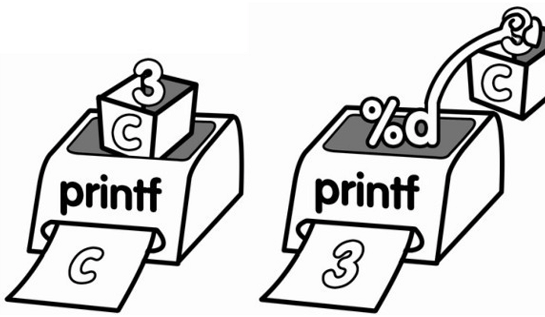Yes, relentlessly to output a c.

So how to output the value of c?

At this time we want to make another role played. “%d” “%d” is actually a “debt collection”, or it can be said to be “beggar”.Its full-time job is “money” to others!Then how should we use it?

``````    printf("%d", c);
``````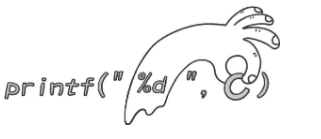Put “% d” between double quotes, put the small house c after the double quotation marks, and use commas. The printf found inside double quotation marks a “debt collection”, will know that at this point need to output a specific value, rather than sign, double quotes to the back of the little house c for the exact value.

Ok, then add the C language code framework, computer to add operation complete code is as follows:

``````#include <stdio.h>
#include <stdlib.h>
int main()
{
int a, b, c;
a=1;
b=2;
c=a+b;
printf("%d", c);
system("pause");
return 0;
}
``````

Now go to give it a try.

## The bedroom for digital - variables

In last section, we learned that computers use to remember one little house Numbers.Computer has many different kinds of small house.

``````  int a;
``````

Represents the apply to the computer for a small house, used to store values, name is a small house.Int and a there is a space between, at the end of a has a semicolon, said the end of the sentence.

If you want to apply for multiple small house, behind a continue with b and c, separated by commas.Such as:

`````` int a, b, c;
``````

There’s a small problem, is to apply for the “little house” name.In principle can literally: can be separate letters, such as a, b or x;Can be a combination of multiple letters, such as aaa, ABC or book;Also can be a combination of letters and Numbers, such as a1 or abc123.Of course, there are some restrictions, if you want to know, please see appendix A.

Here, there may be many students want to ask, just what is meant by the int?

Actually, int control used to store the number of types of small house, said you now apply for small house only integers.

The abbreviation of int is the English word integer (integer).

If you want to put a decimal?

We use a float to apply for a small house, used to store the decimal, form is as follows:

``````float a;
``````

In this way, the small house can be used to store a decimal, such as:

``````float a;
a=1.5;
printf("%f", a);
``````

Means to apply for a small house. A, used to store the decimal in the decimal 1.5.

Note: in C, the decimal is called a floating-point number, using float said.

Before using the printf statements output an integer, using the “% d”.At this time to output decimal, want to use “% f”.

Ok, let’s sum up, the “little house” in our C language terminology is called a variable.Int and float small house is used to store what type of number, we call it here “variable type” or “data types”.

Similar int a;Or float a;Form, we called the “define variables”, their syntax format is as follows:

[English] small house type the name of a small house, the name of a small house; “Term” variable of the type of the variable name, the name of the variable; [code] int a, b; We now know that int a;Said to apply for a small house used to store an integer a, which defines a variable a, to hold the integer.And float a;Said to apply for a small house used to store a decimal a, which defines a floating-point type (solid) variables to store a floating point number (decimal).

Another interesting question, look at the HTML code is as follows:

``````#include <stdio.h>
#include <stdlib.h>
int main()
{
int a;
a=1;
a=2;
printf("%d", a);

system("pause");
return 0;
}
``````

The computer after execution of the code above, please will output 1 or 2?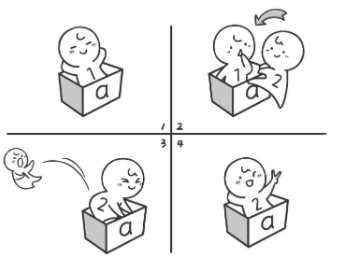## Report problems to us

Hi, if you find something need improved. Please feel free to contact us feedback. We will buy you a coffee if your advise is adopted. Thx.